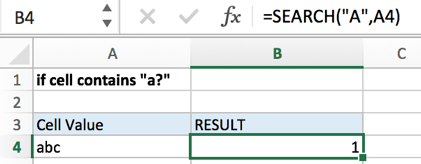# If Cell Contains Certain Text OR Equals Certain Text

IF cell equals certain text

IF function is frequently used in Excel worksheet to return “true value” or “false value” based on the logical test result. If you want to test values to see if they equal certain text like “abc”, you can build formula with IF function to return result.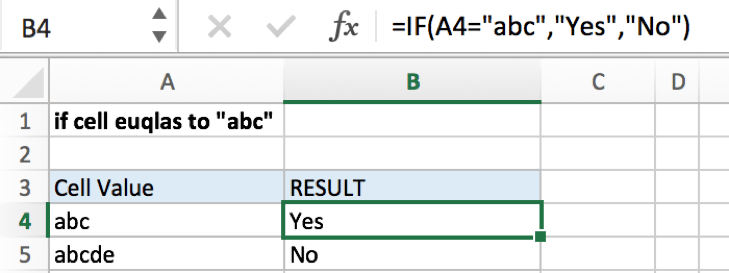## FORMULA

To test if cell equals certain text, the generic formula is:

=IF(A1=”text”,”true value”,”false value”)

Formula in this example

=IF(A4=”abc”,”Yes”,”No”)

## EXPLANATION

In this example, we want to see the results of “if cell equals “abc”” for A4 and A5. Excel IF function can handle this case properly.

IF function allows you to create a logical comparison between your value and reference value (for example “A1>0”), and set true value and false value what you expect to return as test results. IF function returns one of the two results based on logical comparison result.

Syntax: IF(logica_test,[value_if_true],[value_if_false])

To test if A4 equals text “abc”, we can directly create a logical comparison A4=”abc”. “abc” should be quoted by double quotes, if missing double quotes #NAME? error displays instead and it signifies some errors should be corrected in this formula.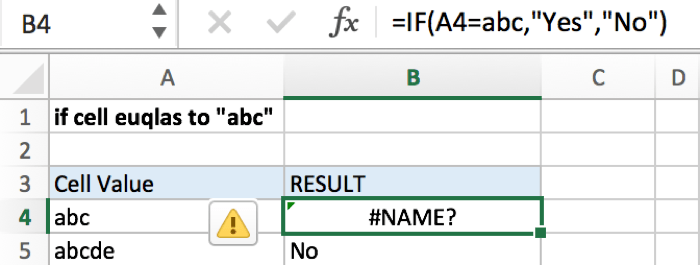We set “Yes” as true result and “No” as false result, A4=”abc” is true, IF evaluates to “Yes”. Cell A5 only contains “abc” not equals “abc”, so it fails logical comparison and get a result of “No”.

IF cell contains certain text

To test if cell contains certain text like “abc”, we can use IF function together with ISNUMBER and SEARCH functions.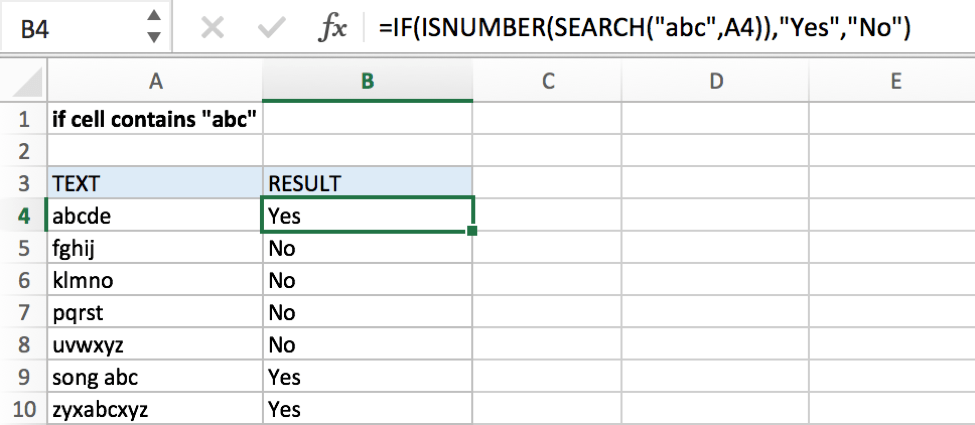## FORMULA

To test if cell contains certain text, the generic formula is:

=IF(ISNUMBER(SEARCH(“text”,A1)),”TRUE”,”FALSE”)

Formula in this example:

=IF(ISNUMBER(SEARCH(“abc”,A4)),”Yes”,”No”)

## FUNCTION INTRODUCTION

SEARCH function can help us to see if text string A (for example “abc”) is included in another text string B (for example “abcde”), and if yes, it returns the position of the first character of the text string B.

Syntax: SEARCH(find_text,within_text,[start_number])

ISNUMBER function returns TRUE if cell contains a number, and returns FALSE if not. This function is easy to understand by its name “ISNUMBER”.

Syntax: ISNUMBER(value)

## EXPLANATION

In this example, we want to know if cells in A column contain text “abc”, so we use SEARCH function to search text “abc” from cells in A column. For example, to test if A4 contains “abc”, we can build formula SEARCH(“abc”,A4), it is equivalent to SEARCH(“abc”,”abcde”).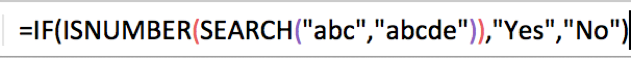After calculating SEARCH function, its result is delivered to ISNUMBER function as test value. In this example “abc” is found in “abcde”, and character “a” is located in the first position of all five characters, so SEARCH returns “1”, ISNUMBER(SEARCH(“abc”,”abcde”))->ISUNUMBER(1). In Excel, ISNUMBER+SEARCH can help us check if specific substring is including in string, or specific text is included in one sentence.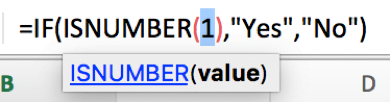Formula ISNUMBER(SEARCH()) is within IF function as argument “logical_test”. Now, ISNUMBER(1) evaluates to “TRUE” and deliver “TRUE” to IF function as logical test result directly.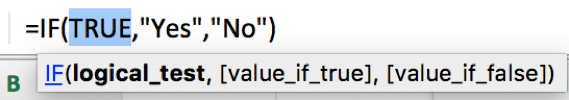IF function returns result of true value “Yes”. For A5 “fghij”, it doesn’t contain “abc”, so IF returns “No” in B5.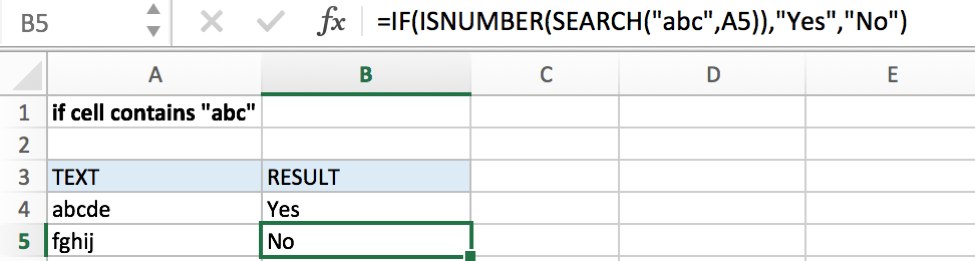## COMMENT

1. SEARCH function supports wildcards like “?” and “*”.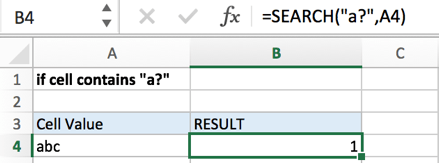1. SEARCH function is case-insensitive.
2. IF function doesn’t support wildcards.

### Related Functions

• Excel IF function
The Excel IF function perform a logical test to return one value if the condition is TRUE and return another value if the condition is FALSE. The IF function is a build-in function in Microsoft Excel and it is categorized as a Logical Function.The syntax of the IF function is as below:= IF (condition, [true_value], [false_value])….
• Excel ISNUMBER function
The Excel ISNUMBER function returns TRUE if the value in a cell is a numeric value, otherwise it will return FALSE.The syntax of the ISNUMBER function is as below:= ISNUMBER (value)…
• Excel SEARCH function
The Excel SEARCH function returns the number of the starting location of a substring in a text string.The syntax of the SEARCH function is as below:= SEARCH  (find_text, within_text,[start_num])…